# Tie in selecting row and column (Vogel's Approximation Method - VAM) | Numerical | Solving Transportation Problem | Transportation Model

## Solve the following problem for BFS using VAM method (Tie in selecting row and column)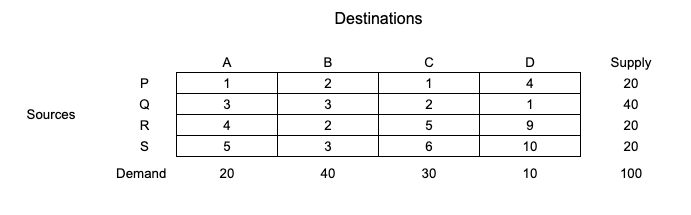### Note: Important point to remember for VAM - Case of Tie

1. If the "smallest cost" in a row or column are repeating, then difference for that row or column is "0".

2. In VAM, we have to select the row or column which is having higher difference

3. But if there is a tie in selection, then we have to select the row or column which contains minimum cost.

4. In case there's a tie in minimum cost too, select the cell in which maximum allocation can be done.

## Solution:

### Step 1: Balance the problem

The given problem is already balanced.

### Step 2: Find row difference and column difference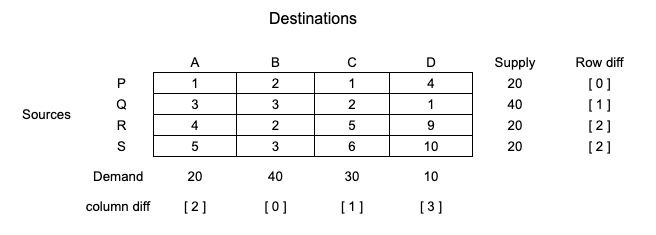### Step 3: Find highest row/column difference and start allocation

→ Select row/column with highest difference. In the same row/column, select the cell with minimum cost, then allocate smallest value of demand or supply in that cell.
→ Here, we have  as the highest difference. Selecting column with  as column difference and finding cell with minimum cost.
→ As we can see here "1" in the second row is the minimum cost in this last column with highest difference of .
→ So, allocating 10 to "1"(min. cost) with highest column difference.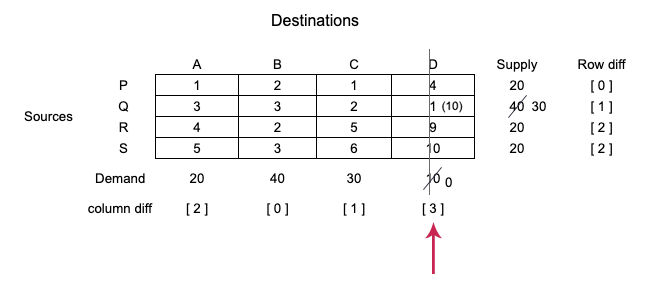### Step 4: Remove the row/column as per fulfillment of supply or demand

→ Remove the row/column whose supply or demand is fulfilled and prepare new matrix as shown below.
→ Check that here, we have multiple highest difference as "".
[Tie] We have to select the row/column which has minimum cost included. (check note above for this step).
→ So, selecting first column with highest column difference as  and minimum cost as "1" and allocating same as we have done in third step above.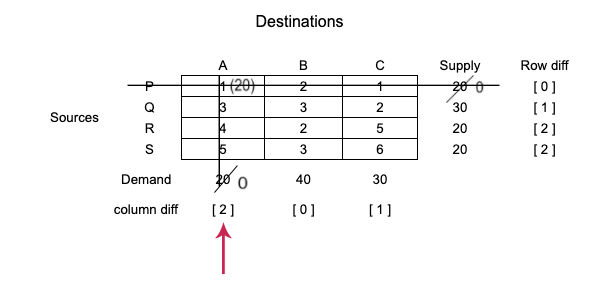### Step 5: Repetition of above steps

→ Repeat the procedure until all allocations are done.
→ You may get [Tie] again in this and further steps. Just repeat step 4 & 3 as above shown to get the answer.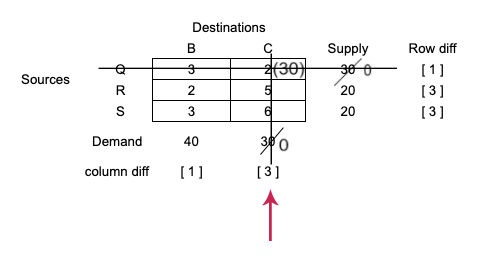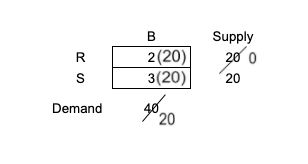### Step 6: After all allocations are done, write the allocations and find transportation cost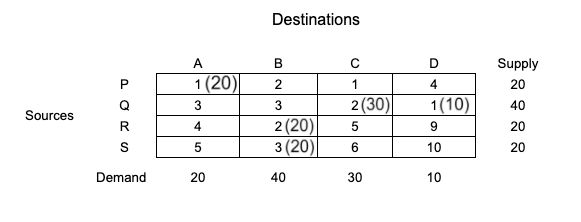\begin{aligned} &Transportation \ Cost\\ &= (1 \times 20) + (2 \times 30) + (1 \times 10) + (2 \times 20) + (3 \times 20)\\ &= 190 \end{aligned}